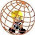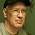"Some say they see poetry in my paintings; I see only science." -Georges Seurat

## Thursday, July 31, 2014

### One Simple Counter to Zeno's Dichotomy Paradox

Zeno was a Greek philosopher who has famously vexed great minds for thousands of years with his puzzling paradoxes that seem to defy explanation or refutation. The problems he describes are typically simple to state, and to understand, but defiantly difficult to deconstruct or disprove. Aristotle, Bertrand Russell, St. Thomas Aquinas, and scores of other heavy-weight philosophers have chimed in with suggested solutions to at least some of Zeno's riddles. I've looked at a lot of these counterarguments, solutions, and refutations to Zeno's Paradoxes, but I've never seen one particular counterargument that seems the most obvious to me.

Zeno is famous for posing at least three major paradoxes: (1) Achilles & the Tortoise; (2) the Arrow Paradox; and (3) the Dichotomy Paradox. It is this last problem to which I am referring. The Dichotomy Paradox is a thorny little thought experiment involving motion of an object, and our mental and mathematical conceptualizations of that motion. The Dichotomy Paradox sneakily pits math versus reality in this little gem of a riddle.

Zeno asks us to consider a person traveling from one city to another, say Athens to Rome. In order for the traveler to go the full distance, d, the traveler must first complete half of the trip, d/2. In order to go the last half, the traveler must again travel half of the remaining distance, so (d/2)/2 = d/4. Zeno argues that the traveler must keep traveling half the distance, then half of the remaining distance, then half of that remaining distance, and so on for infinity, never quite reaching the endpoint. And since no one can travel an infinity of distances in a finite amount of time, Zeno concludes that it is impossible for the traveler to complete his trip by moving! Ergo, motion is just an illusion.

Obviously, people and objects can and do move in the world, so we know from the start that Zeno's conclusion cannot be true, but that's what is so puzzling about it. It was not immediately obvious to philosophers at the time where exactly this line of thinking was going astray.  Most critiques of this Paradox rely on more modern thinking and mathematical techniques that were not available in Zeno's day; namely, infinite sums in which a finite answer is produced. So a traveler going on the number line from zero to one, using Zeno's traveling method of going half, then half again, then half again and again, results in an infinite sum that eventually results in completion of the trip:

0 + 1/2 + 1/4 + 1/8 + 1/16 + 1/32 + ..... = 1

But you don't need to know about infinite sums to dismiss Zeno's Paradox straight away, you just need to point out that he is begging the question (assuming his own conclusion) in this logic, or contradicting himself -- I'm not exactly sure which. I've never actually seen any other philosophers point out this glaring hole in Zeno's logic, so I decided to post my thoughts here in the hopes of being corrected. Let's work backwards, very quickly, which clearly suggests a problem with his thinking:

Zeno concludes traveling the distance d is impossible (or illusory?). But then assumes traveling the distance d/2 is possible. So instead of setting our target distance at d, and never quite reaching it because of the infinite half-sums involved, let's set our target distance to 2 times d = 2d. Since we can apparently travel half-distances no problem, according to Zeno himself, then half of 2d is just d, the distance we wanted to travel in the first place.

Boom, that's it. Problem solved, as far as I can tell. We just showed that traveling distance d was possible using Zeno's own described method of travel, no fancy maths or infinite sums necessary to banish this riddle. Dichotomy Paradox solved?

1.The puzzling part is not that we cannot move (that's a nonsensical conclusion). The puzzling part is that there is no last step. You are moving on the number line from zero to one like this:

time t = 0: moving from 0 to 0.5 in 0.5 seconds
time t = 0.5: moving from 0.5 to 0.75 in 0.25 seconds
time t = 0.75: moving from 0.75 to 0.875 in 0.125 seconds
...
There is no step on the list above like

time t = x: moving from x to 1 in ? seconds

So, take all the infinitely many steps on this list, and you still don't cover point 1.

2.Right but my point was that if we can assume that moving any distance is possible (half the distance) in a finite amount of time, then the paradox disappears under its own argument

3.time t = 0: moving from 0 to 1 in 1 second

and there we go. We can use any finite number of steps to reach point 1 like

time t = 0: moving from 0 to 0.5 in 0.5 seconds
time t = 0.5: moving from 0.5 to 1 in 0.5 seconds

The process described in my previous post is also accomplished in a finite amount of time. At t = 1 we have done infinitely many steps, but none of these infinitely many steps is targeting point 1. Zeno's conclusion, that this means, motion is impossible, is arbitrary. My conclusion is, that

time t = 0: moving from 0 to 0.5 in 0.5 seconds
time t = 0.5: moving from 0.5 to 0.75 in 0.25 seconds
time t = 0.75: moving from 0.75 to 0.875 in 0.125 seconds
...

is not a complete description of what is really going on.

4.By the way, there is a way more compelling "motion impossible" argument from Zeno.

If you want to reach 1 you have to reach 0.5 before
If you want to reach 0.5 you have to reach 0.25 before
If you want to reach 0.25 you have to reach 0.125 before
...

Since there is no first step you can take you cannot even start moving.

1.Yes! That one is much more compelling. This one is dismantled via more modern mathematical thinking than was available in Zeno's time: the infinite sum IS mathematically equivalent to the final value 1. An infinity of numbers exist within any finite magnitude.

5.Even after clarifying this there are still some interesting thought experiments left. If we are drawing a line from 0 to 1 in infinitely many steps within one second:

time t = 0: drawing a line segment from 0 to 0.5 in 0.5 seconds
time t = 0.5: drawing a line segment from 0.5 to 0.75 in 0.25 seconds
time t = 0.75: drawing a line segment from 0.75 to 0.875 in 0.125 seconds
...

At which point exactly can we lift the pen in order to finish the line?

6.Zeno presents the argument as a reductio ad absurdum, so the form of the argument is to assume a hypothesis and prove that the hypothesis, when assumed to be true, leads to absurdity. So he can legitimately assume the premise, "motion is real," to prove that this hypothesis leads to a contradiction. You've got the proposed contradiction right (you cannot complete the infinite task), the contradiction encountered thus proving the hypothesis that "motion is real" is false. So he is allowed to assume the premise, to prove it leads to absurdity.

Additional note - in the modern "refutations" of Zeno's paradoxes that use calculus or infinite sums, you still are not resolving Zeno's paradox. Just because the math works, doesn't mean you can complete an infinite task in the physical world. Simply acknowledging that modern math allows that the infinite sum equals 1 does not show you can cross an infinite number of physical spaces - completing an infinite task in physical space/time is not the same as summing an infinite series (which you also cannot actually "do" in real space/time). The dot-dot-dot hides the task, but doesn't complete it!

7.Thanks for the thoughts Jimmy. Isn't there still a contradiction in the argument, in assuming that motion from 0 to 0.5 (or any distance) is possible but the whole trip from 0 to 1.0 is not?

8.Really your post is very informative
http://awarenesspost.blogspot.com
computer Awareness Post
Welcome To computer Awareness Post Here You Can Learn About Computer Studies...